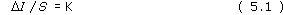Hearing

Start this free course now. Just create an account and sign in. Enrol and complete the course for a free statement of participation or digital badge if available.

Free course

# 9.3.1 Weber's Law

Pioneering work on the relationship between ΔI and S was done by the German physiologist, Ernst Weber in the 1830s. Weber found that the increment in stimulation required for a JND was proportional to the size of the stimulus. Weber had subjects lift a small ‘standard’ weight (S) and then lift a slightly heavier ‘comparison’ (T) weight and judge which was heavier. He found that when the difference between the standard and comparison weights was small, the subjects found it difficult to detect a difference between the weights, but could easily detect large differences – not really suprising. However, he also found that the size of the JND depended on the size of the standard weight. For example, the JND for a 100 g standard weight was 5 g (= ΔI). In other words, the subject could tell the difference between a 100 g standard and a 105 g comparison weight but couldn't tell the difference between a 100 g weight and a comparison weight less than 5 g heavier. In contrast, the JND for a 200 g weight was found to be 10 g (= ΔI); the subject could only detect a difference between a standard 200 g weight and a comparison weight of 210 g or more. Thus, as the magnitude of the stimulus increases, so does the size of the JND.

Research on a number of senses, including hearing, has shown that the JND is larger for larger standard stimuli and that, over a fairly large range of intensities, the ratio of JND to the standard stimulus is constant, i.e.This is called Weber's law which states that the bigger the stimulus, the bigger the increment needed for a change to be detectable. K is called the Weber fraction. Applying the equation to our example of lifted weights, we find that for a 100 g standard, K = 5/100 = 0.05.

## Activity

What does K equal for a 200 g weight?

K=10g/200g=0.05.

Therefore the Weber fraction is constant. What this means is that if I increases, ΔI must increase correspondingly. So, if the standard intensity is low, the increment of change necessary to produce a JND is correspondingly small; by contrast, if the initial intensity is high, the stimulus increment necessary for the JND is correspondingly large.

The Weber fraction holds for most senses, as long as the stimulus intensity is not too close to threshold.